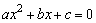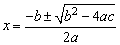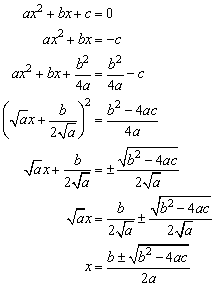## Exams at York: A Few Notes for Overseas Students

Over the past few years, the number of overseas students that have been sitting exams that I’ve been marking has considerably increased, particularly since I've been involved in the new one-year MSc here at York. Since this course has been running, I've noticed two interesting things:

1. In their first term here, the exam scores of the overseas MSc students are significantly lower, on average, than the exam scores of the home students.
2. In the second term, the exam scores of the overseas students are equal to, or even slightly above the exam scores of the home students.
It's difficult to do any controlled experiments to find out why this should be the case, but from talking to students, a few themes consistently appear:
1. The students claim the exams are a “different style to those they have done previously".
2. The students are sometimes not sure what the examiner wants in the answer.

Despite lots of past papers being available, it seems that a lot of students need the shock of doing badly in an exam before they believe that there really is a difference, and they really need to do something about it.

These few notes are designed to help avoid the shock. All feedback very welcome.

### 1) Knowledge is not enough.

We want to see the ability to work with the knowledge, and of your ability to express your opinion, backed by evidence. It seems that some people come from Universities in which the exams are tests of knowledge: provided you learn what's in the notes, you'll be fine.

That's not enough here. You're likely to be asked "why" something works, and to "discuss alternatives". For every fact you come across, ask yourself "why is this true?" If you can't answer that question - do some research, ask the lecturer, read some books. You might need to know.

Don't just learn formulas. Learn when the formula is valid (almost all engineering formulas only work for particular values of their inputs), what assumptions were made to derive the formula (you might be asked how accurate the formula is likely to be).

### 2) You have to think in an exam.

The exam questions will not be the same as in previous year, or as the examples done during the courses.  You have to be able to use the information in the courses to solve new problems.  The best way to learn how to do this is to do as many problems as you can find.  If you run out, make up some new ones and swop them with the other students.  Ask the lecturer if you're unsure.

### 3) Don't panic if you can't do all of the question.

We don't expect anyone to do all of the question correctly. I know that in some countries, 90% is a very good mark on an exam, 80% is a good mark, 70% is average, and below 60% counts as a fail.

It's very different here. It's very, very rare for anyone to get over 90% on an exam, and probably only about 10% of students will score more than 80%. 70% is a very good mark here, 60% is a good mark, and it's only below 50% that you have to worry about failing.

This means that there's no need to do all of the questions to get a very good mark.  If you can do 3/4 of the question and get it right, you'll be one of the top students.

If you think about it, the only way to find out exactly how good someone really is, is to ask them to do something they can't do. That way you find out where their limit is. This means we have to set some problems that we don't expect anyone to be able to do. If you can do them - congratulations - but please don't worry if you can't do everything. That's normal.

Especially important: if you can't do all of a question, or even all of part of a question, don't give up. Tell us what you do know, go as far as you can, write down your thoughts. You'll get marks for any progress you can make. If you write nothing, you'll get nothing.

### 4) Understanding what the questions mean.

For example, consider the words "derive", "explain" and "state". They all mean different things in an exam question, and if you "state" when you should be "deriving" you'll lose marks; and if you "explain" when you should be "stating", you'll be wasting time. Please make sure you know the differences.

For example:
 Keyword Example How to Answer State… State the formula for the roots of a quadratic equation A very short answer is required.  Just write down Derive… Derive the solution to the quadratic equationA long answer is required, start from what you are told in the question, write down every step of the algebra and every assumption you make. …mean What does the term "quadratic equation" mean? All you need to do is define what a quadratic equation is.  There's no need to even mention how to solve it. Explain… Explain what is meant by a "quadratic equation" Same as the previous answer. Comment on… Comment on the formula:Quite a deep question, requiring a long answer.  This includes an explanation of what the formula says, what it does, but also a discussion of when the formula works, when it does not work, and what difficulties there are in using it (e.g. imaginary roots). Discuss.. Discuss quadratic equations Similar to “Comment on…”, but with more scope.  A long answer is required.  You’re free to write anything you know about quadratic equations, including what they are often used for, but you should include all the points under “comment on…” above.

### Example solutions to these questions:

Q) What is meant by a "quadratic equation"?

A) A quadratic equation is any equation that can be expressed in the form.

Q) State the formula for the roots of the quadratic equation:

A)(That's all you need to put for a "state" question. Anything else is a waste of time.)

Q) Derive the formula for the roots of the quadratic equation:

A) By using the word "derive" the examiner is asking you for:Preferably with some comments explained what you're doing. Just writing the solution is not enough - you won't get many marks for that.
For any question that says "derive", start with what information you are given, and work through to the formula, showing all the steps, and clearly stating your assumptions as you go. (For example, the above formula doesn't work when a = 0, so you have to assume that a is not zero.)

Q) Comment on the formulaA) This is the well-known formula that finds the two roots of the quadratic equation. It can be derived by the process of completing the squares, a process that was known to the Babylonians around 400 BCE, and to Chinese mathematicians around 200 years later. The first explicit statement of this equation was made by Brahmagupta in 628 CE. The use of the solutions in the case where b2 - 4ac is negative (and hence the equation has complex roots) was not known until much later. It is derived using the technique of "completing the square".

The formula is not used in this form in numerical algorithms, due to the problems of loss of precision when a and c are much less than b. In this case it is usually better to calculate the larger of the two roots first, and then derive the second root by dividing the ratio c/a by the first root. This indirect approach offers greater accuracy.

and so on... [Thanks to Wikipedia for the information.]

Note that you need to know a lot more about a subject to get good marks on a question that asks you to "comment on" or "discuss" a subject, but there is more of a chance to show off what you do know - if you have a particular interest in history, or computing algorithms, write about them. Either way, you could get good marks here.